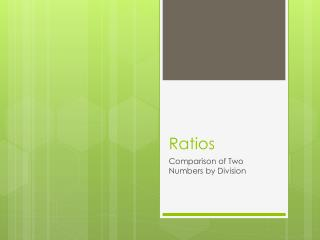DownloadDownload PresentationRatios

# Ratios

Télécharger la présentation## Ratios

- - - - - - - - - - - - - - - - - - - - - - - - - - - E N D - - - - - - - - - - - - - - - - - - - - - - - - - - -
##### Presentation Transcript

1. Ratios Comparison of Two Numbers by Division

2. Ratios • All Fractions are Ratios • A Ratio is a comparison of two numbers by division • There are several ways to write ratios

3. Ways to write ratios • As a fraction (division bar): or • Using the word “to”: 4 to 5 • Using a colon: 4:5 • Using “out of”: 4 out of 5 • All decimals and percents are forms of ratios: “40% chance of rain”

4. Two Types of Ratios • Part to Whole • When a piece of something is compared to all of something • Can be written using all four methods of writing ratios • Part to Part • When a piece of something is compared to the another piece of that something • Can only be written using “to” and the colon

5. If there are 18 ice cream cones and 7 are chocolate, the ratio of chocolate to total is:

6. A ratio is a comparison of two numbers by. . .